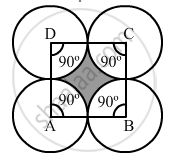Advertisement Remove all ads

# Four Equal Circles Are Described About the Four Corners of a Square So that Each Touches Two of the Others, as Shown in the Figure. Find the Area of the Shaded Region, If Each Side of the - Mathematics

Sum

Four equal circles are described about the four corners of a square so that each touches two of the others, as shown in the figure. Find the area of the shaded region, if each side of the square measures 14 cm.Advertisement Remove all ads

#### Solution

Side of the square = 14 cm
Radius of the circle =14/2 = 7 "cm"
Area of the quadrant of one circle = 1/4pi"r"^2

=1/4xx22/7xx7xx7

= 38.5 cm

Area of the quadrants of four circles = 38.5 × 4 = 154 cm

Now,
Area of the square =(Side)

= 14

= 196 cm

Area of the shaded region = Area of the square -- Area of the quadrants of four circles

= 196 - 154

= 42 cm

Is there an error in this question or solution?
Advertisement Remove all ads

#### APPEARS IN

RS Aggarwal Secondary School Class 10 Maths
Chapter 18 Area of Circle, Sector and Segment
Exercise 18B | Q 36 | Page 833
Advertisement Remove all ads

#### Video TutorialsVIEW ALL 

Advertisement Remove all ads
Share
Notifications

View all notifications

Forgot password?